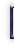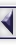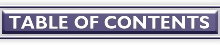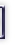# Compiler Design with Python

## 1.1 Purpose

C is obviously the first choice for anybody interested in designing a production quality compiler or interpreter. But what about designing a `little language' just for the fun of it (or maybe, for more serious purposes)? Why worry when you have Python - and some really smart tools to go with it!

## 1.2 The toolkit

We will be using Python Lex-Yacc (PLY) for recognizing tokens and parser construction. These tools are very closely modeled after traditional lex/yacc. If you know how to use these tools in C, you will find PLY to be similar. You can download PLY from the site system.cs.uchicago.edu.ply.

We will need the modules lex.py and yacc.py in our working directory. Also we require Python version 2.1 or higher.

## 2. Getting started

Before going into the details of implementation, let us get down to the basics.

## 2.1 Tokens

What are tokens ? Tokens are symbols like +, -, * or /; or words such as begin, end, if or while - which we would like to identify as operands, reserved words, keywords etc. Tokens must be defined as regular expressions.

## 2.2 Defining the Language

Since we are writing a compiler for a particular language with constructs that we would like to include, the first thing to do is to define the language. This is done by writing a set of rules or grammar for the particular language. For example, if you want your language to provide the 'if-then-else-endif' construct, then one simple way to write a rule for it is :

```     if_statement : IF LPAREN statement RPAREN multiple-statements ELSE
multiple-statements ENDIF
```

where (1) IF, LPAREN, RPAREN, ELSE and ENDIF are tokens for recognizing `if` , `(` , `)` , `else` and `endif` respectively. (2) 'statement' and 'multiple-statements' are again different constructs for which rules are written.

## 2.3 Parsing

In simple terms, parsing is the method of verifying whether the input program does match the rules given to the parser. There are different types of parsing methods. But we needn't go into the details involved. It is only sufficient to know that, given a set of rules (as seen in the example above) the parser sees, if the input constructs corresponds to the rules defined.

## 3. Implementation

Well, we are now ready to implement a compiler. There are different phases of a compiler like token recognition, parsing, taking semantic actions, producing intermediate code, optimizing it, and finally producing the required output assembly code. The steps that we are taking will also be quite similar.

## 3.1 Defining the Language

As said earlier, the first step is to define the language which you want your compiler to accept. You should be certain which constructs and operators you want to provide. Constructs such as 'while', 'if', 'assignment statements' etc are common. So are operands such as +, -, *, / etc. You should write down the rules for your language. A set of rules for a language accepting assignment statements are given below.

```assign_statement    : VAR EQUALS statement
| statement SUBOP term
| term

term                : term MULOP factor
| term DIVOP factor
| factor

factor              : VAR
| NUM
| LPAREN statement RPAREN
```

Throughout our discussion, we adopt the convention that words in upper cases (NUM, VAR, EQUALS, ADDOP, SUBOP, MULOP, DIVOP, LPAREN, RPAREN) are tokens and those in lower cases (assign_statement, statement, term, factor) are rules.

## 3.2 Token Definition and Recognition

Next, we have to define the tokens that we are using. In our example, we have used nine tokens - NUM, VAR, EQUALS, ADDOP, SUBOP, MULOP, DIVOP, LPAREN and RPAREN. The following program implements a simple lexer for tokenizing our language. [text version]

```import lex

# List of token names. This is compulsory.
tokens = (
'NUM',
'VAR',
'EQUALS',
'SUBOP'
'MULOP',
'DIVOP',
'LPAREN',
'RPAREN'
)

# Regular statement rules for tokens.
t_VAR           = r'[a-zA-Z_][\w_]*'
t_EQUALS        = r'='
t_SUBOP         = r'-'
t_MULOP         = r'\*'
t_DIVOP         = r'/'
t_LPAREN        = r'\('
t_RPAREN        = r'\)'

# A regular statement rule with some action code.
def t_NUM(t) :
r'\d+'
try:
t.value = int(t.value)
except ValueError:
print "Line %d: Number %s is too large!" % (t.lineno, t.value)
t.value = 0
return t

# Define a rule so that we can track line numbers.
def t_newline(t):
r'\n+'
t.lineno += len(t.value)

# A string containing ignored characters (spaces and tabs).
t_ignore  = ' \t'

# Error handling rule
def t_error(t):
print "Illegal character '%s'" % t.value
t.skip(1)

# Build the lexer
lex.lex()

# Get the input
data = raw_input()

lex.input(data)

# Tokenize
while 1 :
tok = lex.token()
if not tok :
break
print tok

If you want to include reserved words, it is usually
easier to just match a variable name (identifier) and do a special
name lookup in a function like this:

reserved = {
'if' : 'IF',
'then' : 'THEN',
'else' : 'ELSE',
'while' : 'WHILE',
...
}

def t_VAR(t):
r'[a-zA-Z_][\w_]*'
t.type = reserved.get(t.value,'ID')    # Check for reserved words
return t
```

## 3.3 Parsing

Parsing is quite easy when we use yacc.py. The parser invokes the lexer for getting tokens. So we have to import the lex module that we had written earlier. Now, corresponding to each rule, we define a function and write the rule itself as a document. Within the function we can write the semantic actions needed to be taken. For our example language, the parsing can be done as shown below. [text version]

```# Yacc example

import yacc

# Get the token map from the lexer that we defined
# earlier.  This is required.

from ourexlex import tokens

__var_names = {}

def p_assign_statement(t) :
'assign_statement : VAR EQUALS statement'
__var_names[t] = t

def p_statement_plus(t) :
t = t + t

def p_statement_minus(t) :
'statement : statement SUBOP term'
t = t - t

def p_statement_term(t) :
'statement : term'
t = t

def p_term_times(t) :
'term : term MULOP factor'
t = t * t

def p_term_div(t) :
'term : term DIVOP factor'
t = t / t

def p_term_factor(t) :
'term : factor'
t = t

def p_factor_num(t) :
'factor : NUM'
t = t

def p_factor_var(t) :
'factor : VAR'
if __var_names.has_key(t) :
t = __var_names[t]
else :
print "Undefined Variable", t, "in line no.", t.lineno(1)

def p_factor_expr(t):
'factor : LPAREN statement RPAREN'
t = t

# Error rule for syntax errors
def p_error(t):
print "Syntax error in input!"

# Build the parser
yacc.yacc()

while 1:
try:
s = raw_input('enter > ')
except EOFError:
break
if not s: continue
yacc.parse(s)
```

Here each function accepts a single argument, t, which is a tuple. The values of t[i] are mapped to grammar symbols as shown here:

```
def p_statement_plus(t):
#   ^            ^        ^    ^
#  t         t     t t

t = t + t
```

## 3.4 Semantic actions

The semantic actions are the steps that the parser takes when it reduces the input to a particular rule. In our example above, the actions correspond to that of an interpreter. For a simple compiler, the semantic action may be to produce the assembly code corresponding to a rule.

Suppose you want to produce 8086 assembly instructions as output. Let us assume that 'bx' is a temporary register. Now, whenever we see an operand, we store the contents of the accumulator in the temporary register, and store the operand itself in the accumulator. Thus, the last seen operand (or the result of an evaluation) will always be in the accumulator.

```
def p_factor_num(t) :
'factor : NUM'
__output_fp.write("\tmov bx,ax\n"%f)      # bx <-- [ax]
__output_fp.write("\tmov ax,0x%x\n"%t) # ax <-- t

where, '__output_fp' is a file pointer to an output file

```

Since the operands of an operation (be it binary or unary) is now available, we can write the semantic action for adding as :

```def p_statement_plus(t) :
# ax <-- [ax] + [bx]
```

Similarly, whenever we see a new variable, we can allocate a register for the variable (a stack location is a better choice to store local variables), and remember the register allocated by using a dictionary. The variable name is the key and the register name is the value. Every time a variable name is referenced, the dictionary is searched using the name of the variable as key, to get the corresponding register name.

## 3.5 Optimization

For a C compiler, the assembly instructions are not produced so early as we have depicted here. Actually, it is the intermediate code that is produced. Then the intermediate code is optimized and finally the assembly code is generated.

Since, optimization is itself a vast topic, we will only discuss a simple optimization technique, namely peephole optimization. The easiest way to implement peephole optimization is to hand-code a particular assembly program and compare it with the code your compiler produces.

For example, if your assembly instruction set does not have an instruction for multiplying, then you can make your compiler produce code for multiplication by repetitive addition. A simple optimization that you can make here is this : if you have one operand as 1, then you can store the other operand as the result; instead of going for the repetitive addition, which will obviously be a loop. Again, since the multiplier determines the loop count, you can choose the lower of the two operands as the multiplier.

Another example for peephole optimization is in the use of jump's. Look at the following example :

```
jmp .L1
.
.
.
.
.
.L1         jmp .L2
.
.
.
.
.

```

Here, the first jump statement can be changed to reduce the number of jumps, as is shown below.

```

jmp .L2
.
.
.
.
.
.L1         jmp .L2
.
.
.
.
.
```

There are various algorithms for producing optimized codes. The methods discussed above are only the beginning steps towards complex time and space saving optimization techniques.

## 4. What next ?

The illustration that we have gone through is not a full-fledged compiler. To complete it, we need to implement more and more common constructs. It's only a matter of writing rules for the constructs, defining regular statement for every new token, writing parser functions for the grammar, and finally taking semantic actions in those functions.

####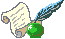Dinil Divakaran

I am a final year computer science student at GEC Thrissur, Kerala, in India.

##### Copyright © 2002, Dinil Divakaran. Copying license http://www.linuxgazette.net/copying.html Published in Issue 79 of Linux Gazette, June 2002